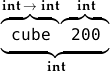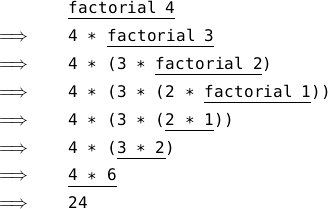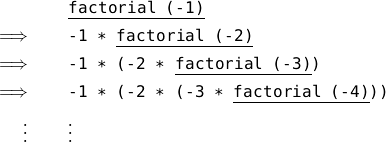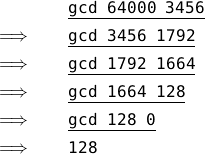Back to Contents

# Names and Functions

So far we have built only tiny toy programs. To build bigger ones, we need to be able to name things so as to refer to them later. We also need to write expressions whose result depends upon one or more other things.

Before, if we wished to use a sub-expression twice or more in a single expression, we had to type it multiple times:

` OCaml`

`# 200 * 200 * 200;;`
`- : int = 8000000`

Instead, we can define our own name to stand for the result of evaluating an expression, and then use the name as we please:

` OCaml`

`# let x = 200;;`
`val x : int = 200`
`# x * x * x;;`
`- : int = 8000000`

To write this all in a single expression, we can use the `let`  …  `=`  … `in`  construct:

` OCaml`

`# let x = 200 in x * x * x;;`
`- : int = 8000000`
`# let a = 500 in (let b = a * a in a + b);;`
`- : int = 250500`

We can also make a function, whose value depends upon some input (we call this input an argument – we will be using the word “input” later in the book to mean something different):

` OCaml`

`# let cube x = x * x * x;;`
`val cube : int -> int = <fun>`
`# cube 200;;`
`- : int = 8000000`

We chose `cube` for the name of the function and `x` for the name of its argument. When we typed the function in, OCaml replied by telling us that its type is int int. This means it is a function which takes an integer as its argument, and, when given that argument, evaluates to an integer. To use the function, we just write its name followed by a suitable argument. In our example, we calculated 2003 by giving the `cube` function `200` as its argument.

The `cube` function has type int int, we gave it an integer `200`, and so the result is another integer. Thus, the type of the expression `cube 200` is int – remember that the type of any expression is the type of the thing it will evaluate to, and `cube 200` evaluates to `8000000`, an integer. In diagram form:If we try an argument of the wrong type, the program will be rejected:

` OCaml`

`# let cube x = x * x * x;;`
`val cube : int -> int = <fun>`
`# cube` `false``;;`
`Error: This expression has type bool but an expression was expected of type`
` int`

Here is a function which determines if an integer is negative:

` OCaml`

`# let neg x = if x < 0 then true else false;;`
`val neg : int -> bool = <fun>`
`# neg (-30);;`
`- : bool = true`

(We added parentheses to distinguish from `neg - 30`). But, of course, this is equivalent to just writing

` OCaml`

`# let neg x = x < 0;;`
`val neg : int -> bool = <fun>`
`# neg (-30);;`
`- : bool = true`

because `x < 0` will evaluate to the appropriate boolean value on its own – `true` if `x` < 0 and `false` otherwise. Here is another function, this time of type char bool. It determines if a given character is a vowel or not:

` OCaml`

`# let isvowel c =`
`    c = ’a’ || c = ’e’ || c = ’i’ || c = ’o’ || c = ’u’;;`
`val isvowel : char -> bool = <fun>`
`# isvowel ’x’;;`
`- : bool = false`

Notice that we typed the function over two lines. This can be done by pressing the Enter key in between lines. OCaml knows that we are finished when we type `;;` followed by Enter as usual. Notice also that we pressed space a few times so that the second line appeared a little to the right of the first. This is known as indentation and does not affect the meaning of the program at all – it is just for readability.

There can be more than one argument to a function. For example, here is a function which checks if two numbers add up to ten:

` OCaml`

`# let addtoten a b =`
` a + b = 10;;`
`val addtoten : int -> int -> bool = <fun>`
`# addtoten 6 4;;`
`- : bool = true`

The type is int int bool because the arguments are both integers, and the result is a boolean. We use the function in the same way as before, but writing two integers this time, one for each argument the function expects.

A recursive function is one which uses itself. Consider calculating the factorial of a given number – the factorial of 4 (written 4! in mathematics), for example, is 4 × 3 × 2 × 1. Here is a recursive function to calculate the factorial of a positive number. Note that it uses itself in its own definition.

` OCaml`

`# let rec factorial a =`
` if a = 1 then 1 else a * factorial (a - 1);;`
`val factorial : int -> int = <fun>`
`# factorial 4;;`
`- : int = 24`

We used `let rec` instead of `let` to indicate a recursive function. How does the evaluation of `factorial 4` proceed?For the first three steps, the `else` part of the conditional expression is chosen, because the argument `a` is greater than one. When the argument is equal to one, we do not use `factorial` again, but just evaluate to one. The expression built up of all the multiplications is then evaluated until a value is reached: this is the result of the whole evaluation. It is sometimes possible for a recursive function never to finish – what if we try to evaluate `factorial (-1)`?The expression keeps expanding, and the recursion keeps going. Helpfully, OCaml tells us what is going on:

` OCaml`

`# let rec factorial a =`
` if a = 1 then 1 else a * factorial (a - 1);;`
`val factorial : int -> int = <fun>`
`# factorial (-1);;`
`Stack overflow during evaluation (looping recursion?).`

This is an example of an error OCaml cannot find by merely looking at the program – it can only be detected during evaluation. Later in the book, we will see how to prevent people who are using our functions from making such mistakes.

One of the oldest methods for solving a problem (or algorithm) still in common use is Euclid’s algorithm for calculating the greatest common divisor of two numbers (that is, given two positive integers a and b, finding the biggest positive integer c such that neither a/c nor b/c have a remainder). Euclid was a Greek mathematician who lived about three centuries before Christ. Euclid’s algorithm is simple to write as a function with two arguments:

` OCaml`

`# let rec gcd a b =`
` if b = 0 then a else gcd b (a mod b);;`
`val gcd : int -> int -> int = <fun>`
`# gcd 64000 3456;;`
`- : int = 128`

Here is the evaluation:Finally, here is a simple function on boolean values. In the previous chapter, we looked at the `&&` and `||` operators which are built in to OCaml. The other important boolean operator is the `not` function, which returns the boolean complement (opposite) of its argument – `true` if the argument is `false`, and vice versa. This is also built in, but it is easy enough to define ourselves, as a function of type bool bool.

` OCaml`

`# let not x =`
` if x then false else true;;`
`val not : bool -> bool = <fun>`
`# not true;;`
`- : bool = false`

Almost every program we write will involve functions such as these, and many larger ones too. In fact, languages like OCaml are often called functional languages.

## Questions

1. Write a function which multiplies a given number by ten. What is its type?

2. Write a function which returns `true` if both of its arguments are non-zero, and `false` otherwise. What is the type of your function?

3. Write a recursive function which, given a number n, calculates the sum 1 + 2 + 3 + … + n. What is its type?

4. Write a function `power x n` which raises `x` to the power `n`. Give its type.

5. Write a function `isconsonant` which, given a lower-case character in the range `’a’``’z’`, determines if it is a consonant.

6. What is the result of the expression  `let`` x = 1 ``in` `let`` x = 2 ``in`` x + x` ?

7. Can you suggest a way of preventing the non-termination of the `factorial` function in the case of a zero or negative argument?